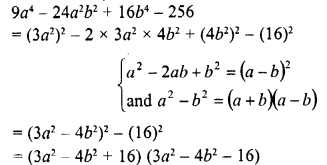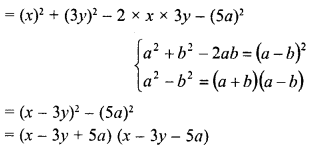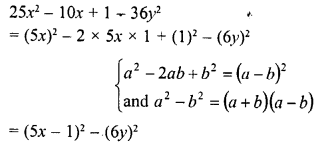# RD Sharma Class 8 Solutions Chapter 7 Factorizations Ex 7.6

In this chapter, we provide RD Sharma Class 8 Solutions Chapter 7 Factorization Ex 7.6 for English medium students, Which will very helpful for every student in their exams. Students can download the latest RD Sharma Class 8 Solutions Chapter 7 Factorization Ex 7.6 Maths pdf, free RD Sharma Class 8 Solutions Chapter 7 Factorization Ex 7.6 Maths book pdf download. Now you will get step by step solution to each question.

### RD Sharma Solutions for Class 8 Chapter 7 Factorization Ex 7.6Download PDF

Factorize each of the following algebraic expressions :
Question 1.
4x2 + 12xy + 9y2
Solution:
4x2 + 12xy + 9y2 = (2x)2 + 2 x 2x x 3y + (3y)2 {∵ a2 + 2ab + b2 = (a +b)2}= (2x + 3y)2

Question 2.
9a2 – 24ab + 16b2
Solution:
9a2 – 24ab + 16b2
= (3a)2 – 2 x 3a x 4b + (4b)2     {∵ a2 – 2ab + b2 = (a – b)2}
= (3a – 4b)2

Question 3.
36a2 – 6pqr + 9r2
Solution:
p2q2 – 6pqr + 9r2
= (pq)2 – 2 x pq x3r + (3r){∵ a2 – 2ab + b2 = (a -b)2}
= (pq-3r)2

Question 4.
36a2 + 36a + 9

Solution:
36a2 + 36a + 9
= (6a)2 + 2 x 6a x 3 + (3)2   {∵ a2 + 2ab + b2 = (a + b)2
= (6a + 3)2

Question 5.
a2 + 2ab + b2 – 16
Solution:
a2 + 2ab + b2 – 16
= (a + b)2 – (4)2     {∵ a2 + 2ab + b2 = (a + b)2 and a2 – b2 = (a + b) (a – b)}
= (a + b + 4) (a + b – 4)

Question 6.
9z2 – x2 + 4xy – 4y2
Solution:
9z2 – x2 + 4xy – 4y2    {∵ a2 – b2 = (a + b) (a – b) and a2 – 2ab + b(a – b)2}
= 9z2 – (x2 – 4xy + 4y2)
= (3z)2 – [(x)2 – 2 x x x 2y + (2y)2]
= (3z)2-(x-2y)2
= (3z + x – 2y) (3z – x + 2y)

Question 7.
9a4 – 24a2b2 + 16b4 – 256
Solution:Question 8.
16 – a6 + 4a3b3 – 4b6
Solution:Question 9.
a2 – 2ab + b2 – c2
Solution:Question 10.
x2 + 2x + 1 – 9y2
Solution:Question 11.
a2 + 4ab + 3b2
Solution:
a2 + 4ab + 3b2
= a2 + 4ab+ 4b2 – b2
= (a)2 + 2 x a x 2b + (2b)2 – b(∵ 3b2 = 4b2 – b2)
= (a + 2b)2 – (b)2   {∵ a2 – b2 = (a +b) (a – b)}
= (a + 2b + b) (a + 2b- b)

Question 12.
96 – 4x-x2
Solution:
96 – 4x – x2 = 96 – (4x + x2)
= 96 – [(x)2 + 2 x x x 2 + (2)2] + (2)2   (on completing the square)
= 96 + 4 – (x + 2)2 = 100 – (x + 2)2
= (10)2 – (x + 2)2
= (10 + x + 2) (10 – x- 2)
= (x + 12) (-x + 8)

Question 13.
a4 + 3a2 + 4
Solution:
a4 + 3a2 + 4
= (a2)2 + (2)2 + 2 x a2 x 2 – a2   (on completing the square)
= (a2 + 2)2 – (a)2
= (a2 + 2 + a) (a2 + 2 – a)
= (a1 + a + 2) (a2 – a + 2)

Question 14.
4a4 + 1
Solution:
4x4 + 1 = (2a2)2 + (1)2 + 2 x 2x2 x 1 – 2 x 2xx 1  (completing the square)
= (2x2 + 1)2 – 4a2
= (2x2 + 1)2 – (2a)2   {a2 – b2 = (a + b) (a – b)}
= (2x2 + 1 + 2a) (2a2 + 1 – 2a)
= (2a2 + 2a + 1) (2a2 – 2a + 1)

Question 15.
4x4+y4
Solution:
4a4 + y4 = (2x2)2 + (y2)2 + 2 x 2x2y2 – 2 x 2x2y2
= (2x2 + y2)2 – 4x2y2
= (2x2 + y2)2 – (2xy)2
= (2x2 + y2 + 2xy) (2x2 + y2 – 2xy)
= (2x2 + 2xy + y2) (2x2 – 2xy + y2)

Question 16.
(x+ 2)2 – 6 (a + 2) + 9
Solution:
(x + 2)2 – 6 (x + 2) + 9
=  (x + 2)2 – 2 x (x + 2) x 3 + (3)2
= (x + 2 – 3)2
= (x-1)2 = (x-1)(x-1)

Question 17.
25 – p2 – q2 – 2pq
Solution:Question 18.
a2 + 9y2 – 6xy – 25a2
Solution:
a2 + 9y2 – 6xy – 25a2Question 19.
49 – a2 + 8ab – 16b2
Solution:Question 20.
a2 – 8ab + 16b2 – 25c2
Solution:Question 21.
x2 -y2+ 6y- 9
Solution:Question 22.
25x2 – 10x + 1 – 36y2
Solution:Question 23.
a2-b2 + 2bc – c2
Solution:Question 24.
a2 + 2ab + b2 -c2
Solution:Question 25.
49 -x2 – y2 + 2xy
Solution:Question 26.
a2 4b2 – 4ab – 4c2
Solution:Question 27.
x2 -y2 – 4xz + 4z
2
Solution:All Chapter RD Sharma Solutions For Class 8 Maths

—————————————————————————–

All Subject NCERT Exemplar Problems Solutions For Class 8

All Subject NCERT Solutions For Class 8

*************************************************

I think you got complete solutions for this chapter. If You have any queries regarding this chapter, please comment on the below section our subject teacher will answer you. We tried our best to give complete solutions so you got good marks in your exam.

If these solutions have helped you, you can also share rdsharmasolutions.in to your friends.